3228.0 - Demographic Estimates and Projections: Concepts, Sources and Methods, 1999
Latest ISSUE Released at 11:30 AM (CANBERRA TIME) 30/08/1999
Page tools:Print AllRSSSearch this Product
Contents >> Appendix 7. The Plus-Minus Proportionate Adjustment technique

A7.1. The Plus-Minus Proportionate Adjustment technique is a procedure for prorating a distribution with positive and negative frequencies. The procedure minimises the adjustments to the original distribution. It requires the use of two factors, one for the positive frequencies and one for the negative frequencies. The formulae are as follows:Adjustment Factor for positive items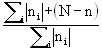Adjustment Factor for negative items :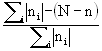where: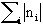= the sum of absolute frequenciesn = the algebraic sum of frequenciesN = the assigned total that the distribution must sum to.A7.2. Having calculated the adjustment factors for positive and negative items, the adjusted values of these items are obtained by multiplying the positive items by the positive factor and the negative items by the negative factor.

A7.3. The technique is illustrated in the following table where the algebraic sum of the frequencies is adjusted from 10 to 15.A7.1: Pro-rata Adjustment of Plus/Minus Frequency Distributioni ni Adjustment factor* Adjusted ni1 30 1.045 312 -50 0.955 -473 30 1.045 31Total 1015Where
n = 10
N = 15
N - n  = 5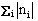= 110

and (*)
Positive Adjustment factor =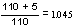Negative Adjustment Factor =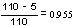Previous Page Next Page